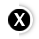## \$115 (USD)1 Algebra: Here to Make Life Simpler

Your first lesson introduces Al-jabru, the Arabic word for algebra that means the science of restoration and canceling. You will search for algebraic solutions and learn definitions, numeric properties, and basic operations that originated from the ancient lands where papyrus was used instead of paper.

2 How Do You Measure Up?

How do calories contribute to weight gain? You will answer this and other questions in this lesson. You will learn the different physical quantities, their units of measurement, and why they're important. You will also learn the difference between mass and weight, and density and weight density.

3 Evaluation Time Again

This lesson focuses on evaluation, but not the type of evaluation your boss might give you. You will learn about algebraic expressions by using the order of operations and the distributive property translation. You will also practice translating word problems into numerical ones.

4 Formula: Another Name for Equation

In this lesson, you will learn formulas for basic shapes: squares and rectangles, triangles, circles, spheres, cylinders, and cubes. You may be thinking, "Is this also a geometry course?" In a way you're correct—some aspects of geometry involve formulas, which people use in everyday life.

5 Balancing Acts: Equations

This lesson will teach you how to balance equations with formulas, such as those used to calculate interest, distance, and temperature. You will also learn how to translate these types of verbal equations into numerical ones. So, this is a chance to hone your mathematics reading skills, too.

6 Investments, Mixtures, and Percentage Changes

In this lesson, you will calculate problems involving percentages, such as how much to invest at different interest rates to obtain optimum interest, and you will learn how to determine a specific quantity of a component of a mixture or solution given specific concentrations.

7 Equate It in Words

The more you practice, the better you will be able to speak the language: the math language. Practice makes perfect! So, in this lesson, you will calculate populations, averages, lengths, and widths. You will also learn how to calculate consecutive integers, including consecutive odd and even integers.

8 Proportions and Variations

In this lesson, you will learn ratios and how to apply them to probability and odds. You will also find out how quantities vary in equations, whether directly or indirectly, and how to apply these variations to scientific laws and equations involving weight, area, and equations of lines.

9 Uniform Linear Motion

This lesson brings you outdoor to solve equations of uniform linear motion, with activities such as jogging, boating, and traveling. You will learn how to calculate distance, rate (speed), and time while going in the same direction, opposite directions, and with wind and water current.

10 Solving Rational Equations

This lesson focuses on using the formula that employs the same concept as the uniform linear motion equation. You will learn to calculate how long it takes to finish a job, how much of the job is done, and at what rate the job is done, depending on the number of people doing the job.

11 Algebraic Rational Equations

Discover some of the natural laws that govern our universe and delve into other physics concepts such as resistance, conductance, focal length, and forces while you learn some history of D-Day.

12  Potpourri of Algebraic Equations

Potpourri, anyone? Test various problem-solving skills while you meet General Sherman and experience the gloominess of the Great Depression.• Learn a new skill or enhance existing skills for professional development or personal enrichment.
• New sessions starting monthly with lessons and assignments released weekly.
• 2-4 hours a week in a convenient six-week format.
• Interactive learning environment. Classroom built around discussion areas where you can engage with classmates and instructors.
• Expert instructors develop, lead, and interact with students in each course.
• Award of completion from your learning institution with passing score.
• Gain the knowledge needed to move forward with your education.
• Start anytime. Access Granted upon registration.
• Courses are designed to be completed within 6-12 weeks.
• Interactive multi-media instruction with integrated assessment, allowing you to work at your own pace.
• Professional instructors support you throughout your learning experience.
• Confirmation of successful course completion.
• Build industry skills or earn continuing education credits in a variety of fields.
• Start Anytime. Access to all course material and assessments from day one.
• Many tutorials can be completed in just a few hours.
• Quick independent study. Learn something new or expand your knowledge while working at your own pace.
• Material developed by industry leaders and student support offered.
• Certificate of completion awarded with passing score.